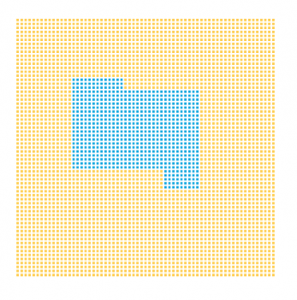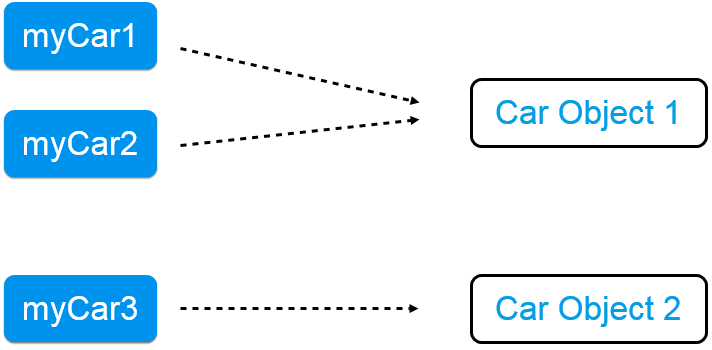# Object Identity and Equality in Java

### This quick tutorial shows you how to compare IDs and values using both the equals operator and the equals() method.

· Java Zone · Tutorial
Save
35.97K Views

## Introduction

In this short article, I will discuss Object Identity and Object Equality in Java.

A PDF of the article is also available here.

## Object Identity

When we create objects in Java, the computer stores them in its memory. To be able to locate an object, the computer assigns it an address in the memory. Every new object you create gets a new address. If this yellow area represents an area of the computer’s memory, the blue area represents our object being stored in the memory. This object will be given some sort of address.Example 1

To illustrate this feature, let us imagine the building featured in Example 2 below. If we are looking at the building, we might be wondering if it is the White House or just another white house object. To check, we can compare this object’s unique address to the White House’s address. We would check our object’s identity using ‘==’, the equals operator. Hopefully the address of that house is “1600 Pennsylvania Avenue North West, Washington DC,” otherwise we’re looking at a different white house object, and the president isn’t waiting inside to meet us.Example 2

Now, let’s declare three variables and discuss their memory locations:

``````Car myCar1 = new Car("blue");
Car myCar2 = myCar1;
Car myCar3 = new Car("blue");``````

Example 3

In Example 3, we have reference variables myCar1, myCar2, and myCar3. myCar1 was assigned a new Car object, as was myCar3, but myCar2 was assigned the value of myCar1. This difference is key. myCar2 is not a new object. It is simply a second reference variable ‘pointing’ to the same object in the memory. So while we have three variables that we created, we actually have only placed two objects in the memory (Example 4).Example 4

Now let’s take these reference variables and compare them using the equals operator, ‘==’. When we use the equals operator, we can see if both variables refer to the same object in the memory. Take a look at the three if statements below:

``````if(myCar1 == myCar1){ // true
}
if(myCar1 == myCar2){ // true
}
if(myCar1 == myCar3){ // false
}``````

Example 5

When we compare myCar1 to itself, it evaluates to true, because they are referring to the same object in the memory. Similarly, myCar1 == myCar2 evaluates to true as well. Again, although they are different reference variables, they are referencing the same object in the memory. Finally, myCar1 == myCar3 evaluates to false, because they are pointing to different objects in the memory.

## Object Equality

Another way that one can to test equality is by using the equals() method. The equals method tells us if two objects are considered equal. Let us suppose that our program requires that two cars are ‘equal’ if they are of the same color. So let’s look at the same three if statements:

``````if(myCar1.equals(myCar1)){ // true
}
if(myCar1.equals(myCar2)){ // true
}
if(myCar1.equals(myCar3){ // false
}``````

Example 6

Based on what you’ve read so far, you’d think that all three statements would evaluate to true. However, that is not how the default equals() method works. If you look at the default equals() method of the Object class, it actually calls ==, giving it the same functionality as simply saying obj1 == obj2.

``````public boolean equals(Object obj) {
return (this == obj);
}``````

Example 7

Obviously, this isn’t what we want. In our example, we want to judge if two Cars are equal based on their color. So, we will have to override the equals() method:

``````@Override
public boolean equals(Object obj) {
if (this == obj) {
return true;
}
if (obj == null) {
return false;
}
if (getClass() != obj.getClass()) {
return false;
}
Car other = (Car) obj;
return this.color.equals(other.color);}

@Override
public int hashCode() {
return color.hashCode();
}``````

Example 8

Now, we are expressing in code what we consider equal or unequal. Again this totally depends on what our client considers equal or unequal. We override these methods not because the creators of Java thought that it would be a good idea, but because there wasn’t any other option. When they wrote the object class, they didn’t really have in mind our car class and the specific way in which we would compare them, so they came up with a generic method that they welcome us to change. You might also notice that I didn’t just overwrite the equals() method. I also overrode the hashCode() method. Java specifies that equal objects must have equal hashCodes as well. For more detail on why we have to override both methods, check out my equals and hashcode article.

Topics:
java, java8, equality, objects

Published at DZone with permission of Marcus Biel, DZone MVB.

Opinions expressed by DZone contributors are their own.